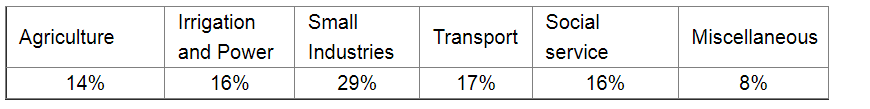# Draw a pie-diagram for the following data of the investment pattern in a five year plan:

Question:

Draw a pie-diagram for the following data of the investment pattern in a five year plan:Solution:

We know:

Central angle of a component $=\left(\right.$ component value $/$ sum of component values $\left.\times 360^{\circ}\right)$

Here the total percentage = 100%

Thus, the central angle for each component can be calculated as follows:

 Item Amount(in %) Sector angle Agriculture 14 14/100 x 360 = 50.4 Irrigation and Power 16 16/100 x 360 = 57.6 Small Industries 29 29/100 x 360 =104.4 Transport 17 17/100 x 360 = 61.2 Social Service 16 16/100 x 360 =57.6 Miscellaneous 8 8/100 x 360 = 28.8

Now, the pie chat representing the given data can be constructed by following the steps below:

Step 1 : Draw circle of an appropriate radius.

Step 2 : Draw a vertical radius of the circle drawn in step 1.

Step 3 : Choose the largest central angle. Here the largest central angle is 104.4o. Draw a sector with the central angle 104.4o in such a way that one of its radii coincides with the radius drawn in step 2 and another radius is in its counter clockwise direction.

Step 4 : Construct the other sectors representing the other items in the clockwise sense in descending order of magnitudes of their central angles.

Step 5 : Shade the sectors with different colours and label them as shown in the figure below.​​